CN：Computer Networks。 Explanation：计算机网络。 Publisher：Elsevier。 SIT： http://dblp.uni-trier.de/db/journals/cn/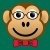0+0+0+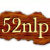0+0+0+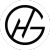0+0+0+0+0+0+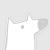0+0+0+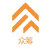@摩点 app还有更多神秘好礼，各位漫威迷们拼手速的时候到了，加油冲鸭！0+0+0+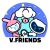#vivo游戏嘉年华# 奇幻V星的观光车马上发车啦，各位团友戳链接报名跟JEEK走：http://t.cn/AigC0vDX 12月21日广州见。0+0+0+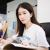0+0+0+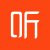#123狂欢节# 【30年后的全民太空旅行】0+0+0+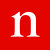0+0+0+0+0+0+0+0+0+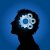0+0+0+0+0+0+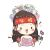0+0+0+
Top